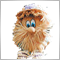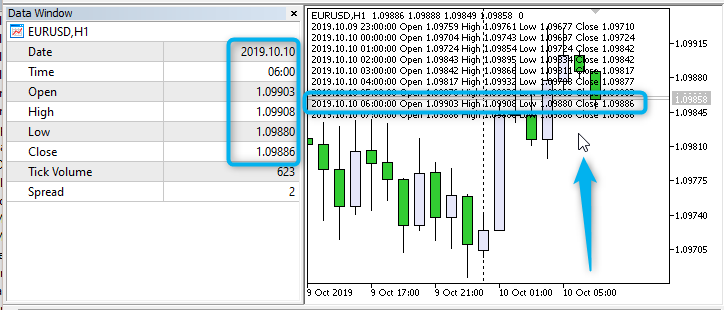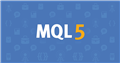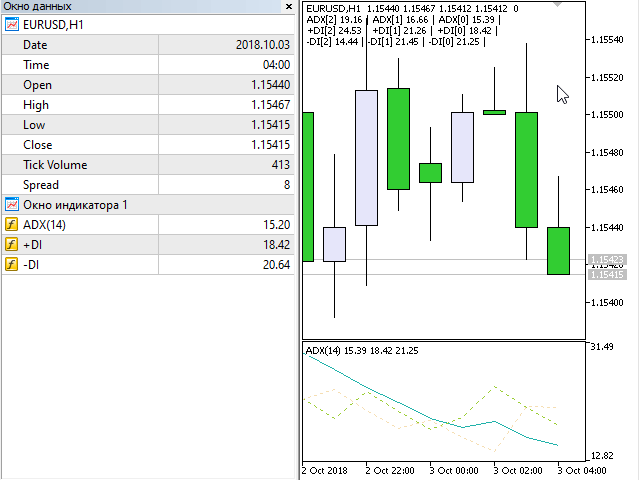104

You need to redo the code: request data not from one bar, but from two. And check the condition not on one bar - but on two.

It was ( ):

Will be:

Thanks. Ive tried editing it but ive almost scattered the entire code. How can i edit this please to add the buy on next bar instead of immediately i.e either at close price of present candle or at new candle open price. Or even to tell it to check the last bar if conditions were met then trigger buy (dont think it would work well but what do i know)

``` if (ask <= Highoo && ask >= low_value && ask<= 10500 )
{
if(rat_value >= current_stoch_low_value && rat_value <= current_stoch_high_value &&
rat_value >= prev_stoch_low_value   && rat_value <= prev_stoch_high_value &&
rsi_value >= rsi_low_value           && rsi_value <= rsi_high_value &&
rsi_value > rsi_value  )

{
set_tp(ticket);
}

}```Moderator
286047

aodunusi:

Thanks. Ive tried editing it but ive almost scattered the entire code. How can i edit this please to add the buy on next bar instead of immediately i.e either at close price of present candle or at new candle open price. Or even to tell it to check the last bar if conditions were met then trigger buy (dont think it would work well but what do i know)

Your explanations are very confusing.  In such cases, I recommend drawing a picture.  In the picture, show the condition for the signal.Moderator
286047

aodunusi :

Thanks. Ive tried editing it but ive almost scattered the entire code. How can i edit this please to add the buy on next bar instead of immediately i.e either at close price of present candle or at new candle open price. Or even to tell it to check the last bar if conditions were met then trigger buy (dont think it would work well but what do i know)

This is how the adviser works now: we check the signal ONLY AT THE MOMENT OF THE BIRTH OF A NEW BAR. The new bar has index # 0, we call the new bar "Current Bar". Example of a BUY signal: if the two previous bars (bar # 1 and bar # 2) are below the '30' line, this is a BUY signal:Pic. 1. iRSI simple advisor, version1.006

The code:

```//+------------------------------------------------------------------+
//| Expert tick function                                             |
//+------------------------------------------------------------------+
void OnTick()
{
//--- we work only at the time of the birth of new bar
datetime time_0=iTime(Symbol(),Period(),0);
if(time_0==m_prev_bars)
return;
m_prev_bars=time_0;
//---
double rsi_2=iRSIGet(2);
double rsi_1=iRSIGet(1);
if(rsi_2==EMPTY_VALUE || rsi_1==EMPTY_VALUE)
return;
//---
if(rsi_2>Inp_RSI_Level_UP && rsi_1>Inp_RSI_Level_UP)
else if(rsi_2<Inp_RSI_Level_DOWN && rsi_1<Inp_RSI_Level_DOWN)
}```
Files:104

This is how the adviser works now: we check the signal ONLY AT THE MOMENT OF THE BIRTH OF A NEW BAR. The new bar has index # 0, we call the new bar "Current Bar". Example of a BUY signal: if the two previous bars (bar # 1 and bar # 2) are below the '30' line, this is a BUY signal:

Pic. 1. iRSI simple advisor, version1.006

The code:

Couldn't get it to work... This is the file for the EA, please help me look at it to understand me.

I want it to check the current bar till the the parameters or conditions are met and once it is it it normally opens a buy but instead I want it to hold that request then trigger the buy on the new candle open or when the current candle ends (still the same thing)

Your EA is checking it bars after bars meaning each bar is showing the average, I'm checking every tick with my EA and once that tick in the current bar fulfils my conditions I want the buy to be triggered on the next bar.

Thanks for the help so far
Files:104

aodunusi:
Couldn't get it to work... This is the file for the EA, please help me look at it to understand me.

I want it to check the current bar till the the parameters or conditions are met and once it is it it normally opens a buy but instead I want it to hold that request then trigger the buy on the new candle open or when the current candle ends (still the same thing)

Your EA is checking it bars after bars meaning each bar is showing the average, I'm checking every tick with my EA and once that tick in the current bar fulfils my conditions I
The buy gets triggered immediately after the signal is received and the signal is usually slow, so I want to delay the buy from the current candle to the next candle after the condition has been met. I.E I am checking the ticks of the former candle and once my condition has been met, I want to delay or hold the buy or sell order to the next bar that opens or when the current bar closes (they are the same thing though)
Files:Moderator
286047

aodunusi :
Couldn't get it to work... This is the file for the EA, please help me look at it to understand me.

I want it to check the current bar till the the parameters or conditions are met and once it is it it normally opens a buy but instead I want it to hold that request then trigger the buy on the new candle open or when the current candle ends (still the same thing)

Your EA is checking it bars after bars meaning each bar is showing the average, I'm checking every tick with my EA and once that tick in the current bar fulfils my conditions I want the buy to be triggered on the next bar.

Thanks for the help so far

In this version: the signal is checked at each tick: as soon as the signal is received, we immediately stop the search for the signal and wait for a new bar. At the time of the birth of a new bar (if there is a saved signal), open a position.

```//+------------------------------------------------------------------+

//+------------------------------------------------------------------+

#property version   "1.007"
//---
//--- input parameters
input int                  Inp_RSI_ma_period       = 14;          // RSI: averaging period
input ENUM_APPLIED_PRICE   Inp_RSI_applied_price   = PRICE_CLOSE; // RSI: type of price
input double               Inp_RSI_Level_UP        = 70;          // RSI Level UP
input double               Inp_RSI_Level_DOWN      = 30;          // RSI Level DOWN
//---
int      handle_iRSI;                           // variable for storing the handle of the iRSI indicator
datetime m_prev_bars                = 0;        // "0" -> D'1970.01.01 00:00';
int      m_signal                   = 0;        // "-1" -> SELL, "0" -> NONE, "1" ->BUY
bool     m_wait                     = false;
//+------------------------------------------------------------------+
//| Expert initialization function                                   |
//+------------------------------------------------------------------+
int OnInit()
{
//--- create handle of the indicator iRSI
handle_iRSI=iRSI(Symbol(),Period(),Inp_RSI_ma_period,Inp_RSI_applied_price);
//--- if the handle is not created
if(handle_iRSI==INVALID_HANDLE)
{
//--- tell about the failure and output the error code
PrintFormat("Failed to create handle of the iRSI indicator for the symbol %s/%s, error code %d",
Symbol(),
EnumToString(Period()),
GetLastError());
//--- the indicator is stopped early
return(INIT_FAILED);
}
//---
return(INIT_SUCCEEDED);
}
//+------------------------------------------------------------------+
//| Expert deinitialization function                                 |
//+------------------------------------------------------------------+
void OnDeinit(const int reason)
{
//---

}
//+------------------------------------------------------------------+
//| Expert tick function                                             |
//+------------------------------------------------------------------+
void OnTick()
{
//---
double rsi_0=iRSIGet(0);
if(rsi_0==EMPTY_VALUE)
return;
//--- we check the signal at each tick
if(!m_wait)
{
if(rsi_0<Inp_RSI_Level_DOWN)
{
m_signal=1; // we remember the BUY signal
m_wait=true;
}
else
{
if(rsi_0>Inp_RSI_Level_UP)
{
m_signal=-1; // we remember the SELL signal
m_wait=true;
}
}
}
//--- we work only at the time of the birth of new bar
datetime time_0=iTime(Symbol(),Period(),0);
if(time_0==m_prev_bars)
return;
m_prev_bars=time_0;

if(m_signal==1)
else
if(m_signal==-1)
//--- we reset the signal
if(m_signal!=0)
{
m_signal=0;
m_wait=false;
}
//---
}
//+------------------------------------------------------------------+
//+------------------------------------------------------------------+
{
//---
int d=0;
}
//+------------------------------------------------------------------+
//| Get value of buffers for the iRSI                                |
//+------------------------------------------------------------------+
double iRSIGet(const int index)
{
double RSI;
//--- reset error code
ResetLastError();
//--- fill a part of the iRSI array with values from the indicator buffer that has 0 index
if(CopyBuffer(handle_iRSI,0,index,1,RSI)<0)
{
//--- if the copying fails, tell the error code
PrintFormat("Failed to copy data from the iRSI indicator, error code %d",GetLastError());
//--- quit with zero result - it means that the indicator is considered as not calculated
return(EMPTY_VALUE);
}
return(RSI);
}
//+------------------------------------------------------------------+
```
Files:Moderator
286047

### Example CopyRates

The first form of calling CopyRates is used:

```int  CopyRates(
string           symbol_name,       // symbol name
ENUM_TIMEFRAMES  timeframe,         // period
int              start_pos,         // start position
int              count,             // data count to copy
MqlRates         rates_array[]      // target array to copy
);```

Please note that ArraySetAsSeries is applied to the ' rates ' array - in this case, rates  corresponds to the rightmost bar on the chart.

```   MqlRates rates[];
ArraySetAsSeries(rates,true);```

The full code:

```//+------------------------------------------------------------------+
//|                                            Example CopyRates.mq5 |
//+------------------------------------------------------------------+
#property version   "1.000"
//--- input parameters
input uchar InpCount = 9; // Data count to copy
//---
datetime m_prev_bars                = 0;        // "0" -> D'1970.01.01 00:00';
//+------------------------------------------------------------------+
//| Expert initialization function                                   |
//+------------------------------------------------------------------+
int OnInit()
{
//---

//---
return(INIT_SUCCEEDED);
}
//+------------------------------------------------------------------+
//| Expert deinitialization function                                 |
//+------------------------------------------------------------------+
void OnDeinit(const int reason)
{
//---

}
//+------------------------------------------------------------------+
//| Expert tick function                                             |
//+------------------------------------------------------------------+
void OnTick()
{
//--- we work only at the time of the birth of new bar
datetime time_0=iTime(Symbol(),Period(),0);
if(time_0==m_prev_bars)
return;
m_prev_bars=time_0;

MqlRates rates[];
ArraySetAsSeries(rates,true);

int start_pos=0,count=(InpCount<1 || InpCount>9)?9:InpCount;
if(CopyRates(Symbol(),Period(),start_pos,count,rates)!=count)
{
m_prev_bars=0;
return;
}

string text="";
for(int i=count-1; i>=0; i--)
{
text=text+
TimeToString(rates[i].time,TIME_DATE|TIME_SECONDS)+
" Open "+DoubleToString(rates[i].open,Digits())+
" High "+DoubleToString(rates[i].high,Digits())+
" Low "+DoubleToString(rates[i].low,Digits())+
" Close "+DoubleToString(rates[i].close,Digits())+"\n";
}
Comment(text);
}
//+------------------------------------------------------------------+
```

Result:Documentation on MQL5: Timeseries and Indicators Access / CopyRates
• www.mql5.com
Gets history data of MqlRates structure of a specified symbol-period in specified quantity into the rates_array array. The elements ordering of the copied data is from present to the past, i.e., starting position of 0 means the current bar. If you know the amount of data you need to copy, it should better be done to a statically allocated...
Files:Moderator
286047

### Example: delete all pending orders of a certain type.

An example in the form of a script: in the input parameter ' Delete all: ' the type of a pending order is set and then the delete function 'DeleteOrders ' is called

```//+------------------------------------------------------------------+
//|                                               Pending Delete.mq5 |
//+------------------------------------------------------------------+
#property version   "1.000"
//---
//---
COrderInfo     m_order;                      // object of COrderInfo class
//---
#property script_show_inputs
//---
//+------------------------------------------------------------------+
//| Enum Pending                                                     |
//+------------------------------------------------------------------+
enum ENUM_PENDING
{
sell_limit=3,  // Sell limit
sell_stop=3,   // Sell Stop
};
//--- input parameters
input ENUM_PENDING   InpPending  = buy_limit; // Delete all:
//+------------------------------------------------------------------+
//| Script program start function                                    |
//+------------------------------------------------------------------+
void OnStart()
{
//---
DeleteOrders((ENUM_ORDER_TYPE)InpPending);
}
//+------------------------------------------------------------------+
//| Delete Orders                                                    |
//+------------------------------------------------------------------+
void DeleteOrders(const ENUM_ORDER_TYPE order_type)
{
for(int i=OrdersTotal()-1; i>=0; i--) // returns the number of current orders
if(m_order.SelectByIndex(i))     // selects the pending order by index for further access to its properties
if(m_order.OrderType()==order_type)
{
continue;
}
}
//+------------------------------------------------------------------+
```

this is the simplest example: there is no filter by symbol, there is no filter by Magic number, there is no verification of the minimum distance (level of freezing), and there is no cycle to guarantee deletion.

Files:Moderator
286047

### Example: Calculate Positions and Pending Orders

Code: Calculate Positions and Pending Orders.mq5

Trade classes CPositionInfo and COrderInfo are used (Do not confuse current pending orders with positions, which are also displayed on the "Trade" tab of the "Toolbox" of the client terminal.)

The code:

```//+------------------------------------------------------------------+
//|                       Calculate Positions and Pending Orders.mq5 |
//+------------------------------------------------------------------+
#property version   "1.001"
//---
//---
CPositionInfo  m_position;                   // object of CPositionInfo class
COrderInfo     m_order;                      // object of COrderInfo class
//+------------------------------------------------------------------+
//| Expert initialization function                                   |
//+------------------------------------------------------------------+
int OnInit()
{
//---

//---
return(INIT_SUCCEEDED);
}
//+------------------------------------------------------------------+
//| Expert deinitialization function                                 |
//+------------------------------------------------------------------+
void OnDeinit(const int reason)
{
//---

}
//+------------------------------------------------------------------+
//| Expert tick function                                             |
//+------------------------------------------------------------------+
void OnTick()
{
//---

Comment(text);
//---
}
//+------------------------------------------------------------------+
//| Calculate all positions Buy and Sell                             |
//+------------------------------------------------------------------+
{
count_sells=0;

for(int i=PositionsTotal()-1; i>=0; i--)
if(m_position.SelectByIndex(i)) // selects the position by index for further access to its properties
//if(m_position.Symbol()==m_symbol.Name() && m_position.Magic()==InpMagic)
{

if(m_position.PositionType()==POSITION_TYPE_SELL)
count_sells++;
}
//---
return;
}
//+------------------------------------------------------------------+
//| Calculate all pending orders                                     |
//+------------------------------------------------------------------+
{
count_sell_limits = 0;
count_sell_stops  = 0;

for(int i=OrdersTotal()-1; i>=0; i--) // returns the number of current orders
if(m_order.SelectByIndex(i))     // selects the pending order by index for further access to its properties
//if(m_order.Symbol()==m_symbol.Name() && m_order.Magic()==InpMagic)
{
else
if(m_order.OrderType()==ORDER_TYPE_SELL_LIMIT)
count_sell_limits++;
else
else
if(m_order.OrderType()==ORDER_TYPE_SELL_STOP)
count_sell_stops++;
}
}
//+------------------------------------------------------------------+
```Documentation on MQL5: Standard Library / Trade Classes / CPositionInfo
• www.mql5.com
Files:Moderator
286047

### A simple example. Displaying iADX indicator values on a chart

This is a simple example: how to get the values of the iADX indicator. For control, the obtained values are displayed on the screen.

Code:

```//+------------------------------------------------------------------+
//|                               Example iADX values on a chart.mq5 |
//+------------------------------------------------------------------+
#property version   "1.00"
//--- input parameters
//---
int    handle_iADX;                          // variable for storing the handle of the iADX indicator
//+------------------------------------------------------------------+
//| Expert initialization function                                   |
//+------------------------------------------------------------------+
int OnInit()
{
//--- create handle of the indicator iADX
//--- if the handle is not created
{
//--- tell about the failure and output the error code
PrintFormat("Failed to create handle of the iADX indicator for the symbol %s/%s, error code %d",
Symbol(),
EnumToString(Period()),
GetLastError());
//--- the indicator is stopped early
return(INIT_FAILED);
}
//---
return(INIT_SUCCEEDED);
}
//+------------------------------------------------------------------+
//| Expert deinitialization function                                 |
//+------------------------------------------------------------------+
void OnDeinit(const int reason)
{
//---

}
//+------------------------------------------------------------------+
//| Expert tick function                                             |
//+------------------------------------------------------------------+
void OnTick()
{
//---
string text="";
ArraySetAsSeries(plus_di,true);
ArraySetAsSeries(minus_di,true);
int start_pos=0,count=3;
{
return;
}

for(int i=count-1; i>=0; i--)
{
text_plus_di   = text_plus_di +"+DI"+"["+(string)i+"]"+" "+DoubleToString(plus_di[i],2)  +" | ";
text_minus_di  = text_minus_di+"-DI"+"["+(string)i+"]"+" "+DoubleToString(minus_di[i],2) +" | ";
}
}
//+------------------------------------------------------------------+
//| Get value of buffers                                             |
//+------------------------------------------------------------------+
bool iGetArray(const int handle,const int buffer,const int start_pos,
const int count,double &arr_buffer[])
{
bool result=true;
if(!ArrayIsDynamic(arr_buffer))
{
PrintFormat("ERROR! EA: %s, FUNCTION: %s, this a no dynamic array!",__FILE__,__FUNCTION__);
return(false);
}
ArrayFree(arr_buffer);
//--- reset error code
ResetLastError();
//--- fill a part of the iBands array with values from the indicator buffer
int copied=CopyBuffer(handle,buffer,start_pos,count,arr_buffer);
if(copied!=count)
{
//--- if the copying fails, tell the error code
PrintFormat("ERROR! EA: %s, FUNCTION: %s, amount to copy: %d, copied: %d, error code %d",
__FILE__,__FUNCTION__,count,copied,GetLastError());
//--- quit with zero result - it means that the indicator is considered as not calculated
return(false);
}
return(result);
}
//+------------------------------------------------------------------+
```

Result:Files: